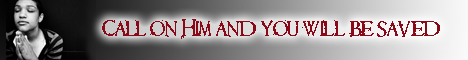Note:  Do not rely on this information. It is very old.

# Acceleration

Acceleration, in hinematics, or science of motion, the rate of change of velocity of a body. That which produces it is termed a force (q.v.), and relates to dynamics. If a body, starting from rest, is subject to a constant acceleration, its velocity at any instant is proportional to the time it has been in motion, and to its acceleration. Change of direction implies change of velocity (q.v.), and therefore implies an acceleration. Hence a body moving along any curve has an acceleration, though it move with constant speed.